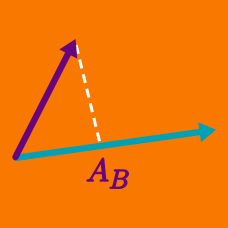Geometry

# Dot Product - Distance between Point and a Line

Find the shortest distance between the point $P=(1,-2,3)$ and the line $2(x-3)=y-1=z+1.$

Find the shortest distance between the point $P=(0,3,4)$ and the line $x=0,\ \frac{y}{2}=-z+3.$

Find the shortest distance between the origin and the line $x-1=\frac{y+1}{-4}=\frac{z-7}{3}.$

What is the shortest distance between the line $x-2=y-1=z+1$ and the sphere $x^2+y^2+z^2=4?$

Find the shortest distance between the origin and the line $\frac{x+1}{2}=-y+2=-z+2.$

×